# Copy the contents of the previous row to the next row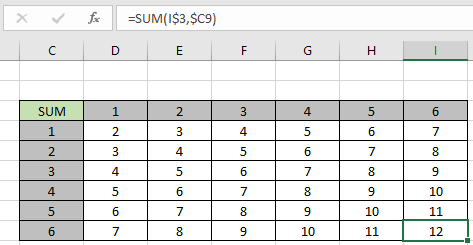In this article, we will learn Copy the contents of the previous row to the next row in Excel

Types of copy and paste methods in Excel ?

In Excel, when you use copy and Paste on any cell. You can do this in many different ways. Like you can just copy the value, formula, format, comments, validation or all at once. But there is one problem with absolute or relative references. When you copy and paste formulas with references, you need to be careful.

Copy and paste with references

Here we have a list of numbers and we need to sum 1 to all the numbers. So I used the formula as shown below.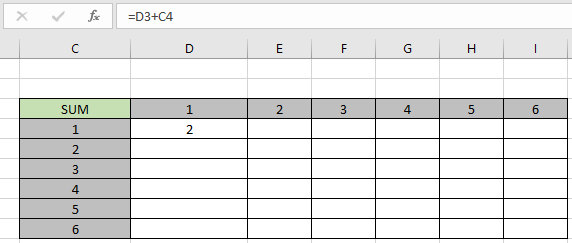Now what do think what happens when i copy the formula and paste to all the other cells.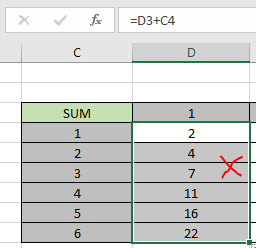As you can see it's not right, as the relative reference gets extended as well. Let's understand this with an example below.

Example :

All of these might be confusing to understand. Let's understand how to use the function using an example. Here we will find the sum of the corresponding values.

Use the formula

=SUM(D\$3,\$C4)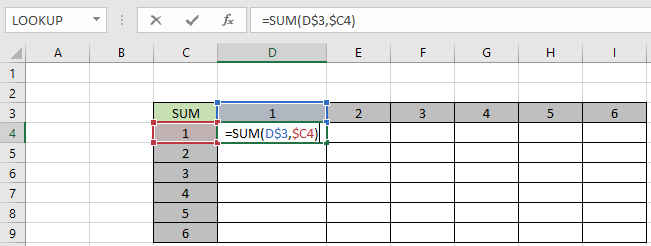Here we need to fix the D3 cell when copying and pasting the formula along the D column and fix the C4 cell when copying and pasting the formula along the row.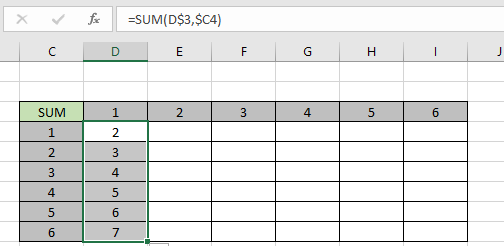As you can see the formula works fine when pasting formulas along the column. Now paste formula along the row to understand its feature.As you can see, the formula works fine for both rows and columns. You can also copy (ctrl + C) and paste (Ctrl + V) to use the same feature.

Here are all the observational notes using the method in Excel
Notes :

1. Use Ctrl + D or drag down option to copy and paste the formula from top most cell to all the selected cells below
2. Use Ctrl + R or the drag right option to copy and paste the formula from the left most cell to all the selected cells on the right.
3. Or use the paste special method to learn more about copying and pasting values in Excel

Hope this article about Copy the contents of the previous row to the next row in Excel is explanatory. Find more articles on copy and paste values and related Excel shortcuts here. If you liked our blogs, share it with your friends on Facebook. And also you can follow us on Twitter and Facebook. We would love to hear from you, do let us know how we can improve, complement or innovate our work and make it better for you. Write to us at info@exceltip.com.

Related Articles:

How to use the Shortcut To Toggle Between Absolute and Relative References in Excel : F4 shortcut to convert absolute to relative reference and same shortcut use for vice versa in Excel.

How to use Shortcut Keys for Merge and Center in Excel : Use Alt and then follow h, m and c to Merge and centre cells in Excel.

How to Select Entire Column and Row Using Keyboard Shortcuts in Excel : Use Ctrl + Space to select whole column and  Shift + Space to select whole row using keyboard shortcut in Excel

Paste Special Shortcut in Mac and Windows : In windows, the keyboard shortcut for paste special is Ctrl + Alt + V. Whereas in Mac, use Ctrl + COMMAND + V key combination to open the paste special dialog in Excel.

How to Insert Row Shortcut in Excel : Use Ctrl + Shift + = to open the Insert dialog box where you can insert row, column or cells in Excel.

Popular Articles:

How to use the IF Function in Excel : The IF statement in Excel checks the condition and returns a specific value if the condition is TRUE or returns another specific value if FALSE.

How to use the VLOOKUP Function in Excel : This is one of the most used and popular functions of excel that is used to lookup value from different ranges and sheets.

How to use the COUNTIF Function in Excel : Count values with conditions using this amazing function. You don't need to filter your data to count specific values. Countif function is essential to prepare your dashboard.

How to Use SUMIF Function in Excel : This is another dashboard essential function. This helps you sum up values on specific conditions.

1.